﻿ 基于Retinex算法的舰船无线传感网络目标跟踪方法
 舰船科学技术2001, Vol. 44Issue (6): 149-152    DOI: 10.3404/j.issn.1672-7649.2022.06.031PDF

1. 金华市现代制造与材料高新技术研发中心，浙江 东阳 322100;
2. 浙江广厦建设职业技术大学，浙江 东阳 322100

Target tracking method of ship wireless sensor network based on retinex algorithm
LIN Chun-lei1,2, PAN Guang-yong1,2
1. Jinhua Modern Manufacturing and Material High-tech Research and Development Center, Dongyang 322100, China;
2. Zhejiang Guangsha Construction Vocational and Technical University, Dongyang 322100, China
Abstract: In order to ensure the reliability of ship wireless sensor network target tracking in different marine environments, a ship wireless sensor network target tracking method based on Retinex algorithm is proposed. Collect the ship target image, use the improved Retinex algorithm to enhance the collected ship target image, improve the image details and quality, transmit the image through wireless communication, convert the target image coordinates to the world coordinate system coordinates according to the camera calibration principle, obtain the actual position of the ship target, and complete the ship wireless sensor network target tracking. The test results show that the edge definition of the enhanced image is improved, the tracking performance is good, and the highest frame rate is about 127 fps; When the ship target is blocked and the scale changes, the target tracking can be completed reliably.
Key words: retinex algorithm     wireless sensor network     target tracking     vision sensor     image enhancement
0 引　言

1 舰船图像无线传感网络目标跟踪方法 1.1 舰船图像无线传感网络目标跟踪方法框架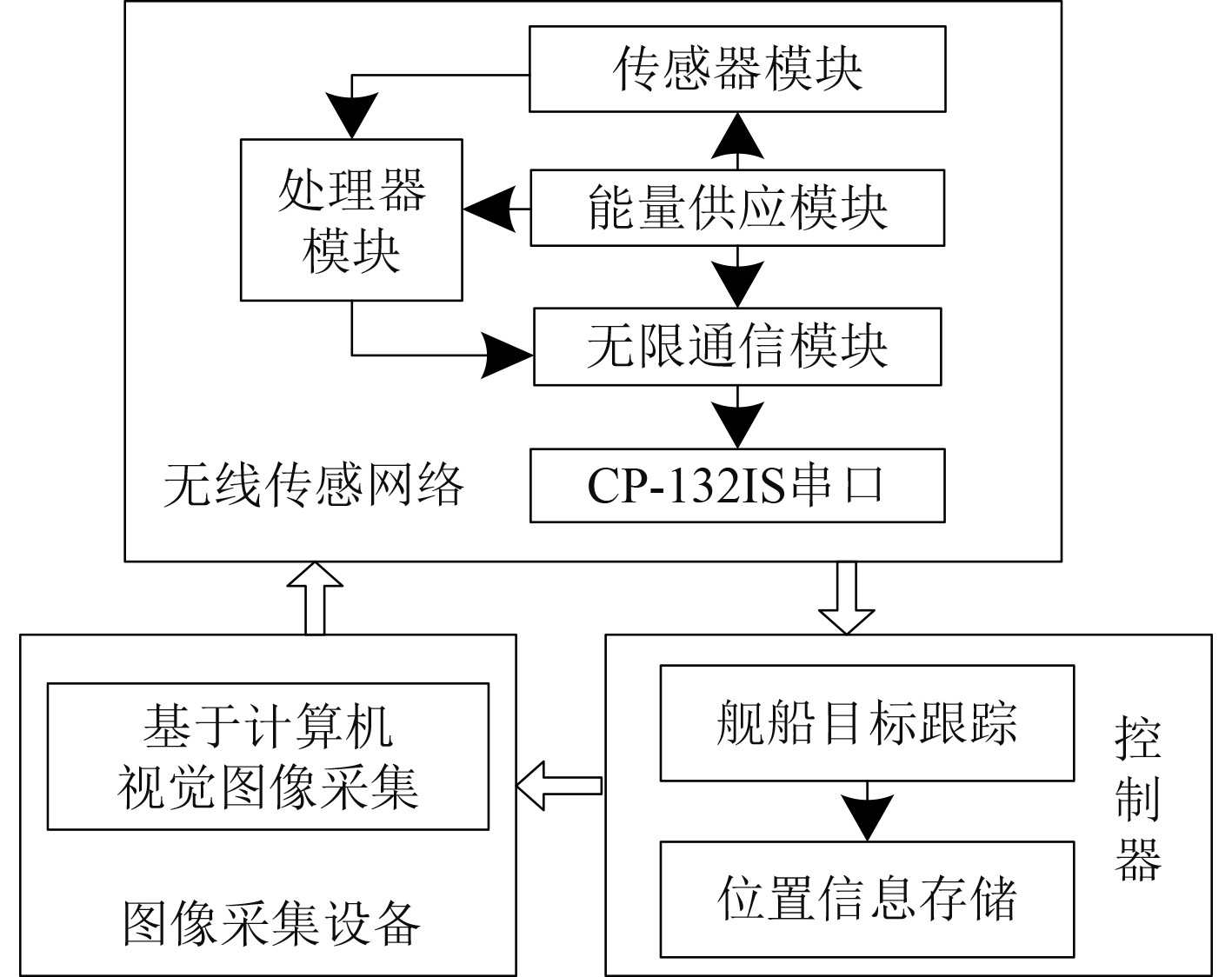图 1 舰船图像无线传感网络目标跟踪方法框架 Fig. 1 Target tracking method framework of ship image wireless sensor network
1.2 基于计算机视觉技术的舰船目标图像采集

1.3 改进Retinex算法的舰船目标图像增强

 $c\left( f \right) = \# \left\{ {\left. p \right|\left| {{f_p} - {f_{p + 1}}} \right| \ne 0} \right\}。$ (1)

1）对I(x,y)实行读取，采用L0范数图像平滑因子完成I(x,y)中R，E，B颜色通道的处理。

2）尺度参数 $\sigma$ 的初始化处理，且 $\lambda '$ $\sigma$ 对应；循环加入L0梯度最小化因子并进行迭代，以此实现I(x,y)不同尺度轮廓的均匀增强。

3）对I(x,y)增强成分实行提取，即获取该图像的轮廓信息，并将其与原始的图像实行融合处理，抑制图像中不重要的图像细节，使图像中的关键细节突出化，实现图像增强，增强后图像为 $I'\left( {x,y} \right)$

1.4 基于摄像头标定的无线传感网络目标跟踪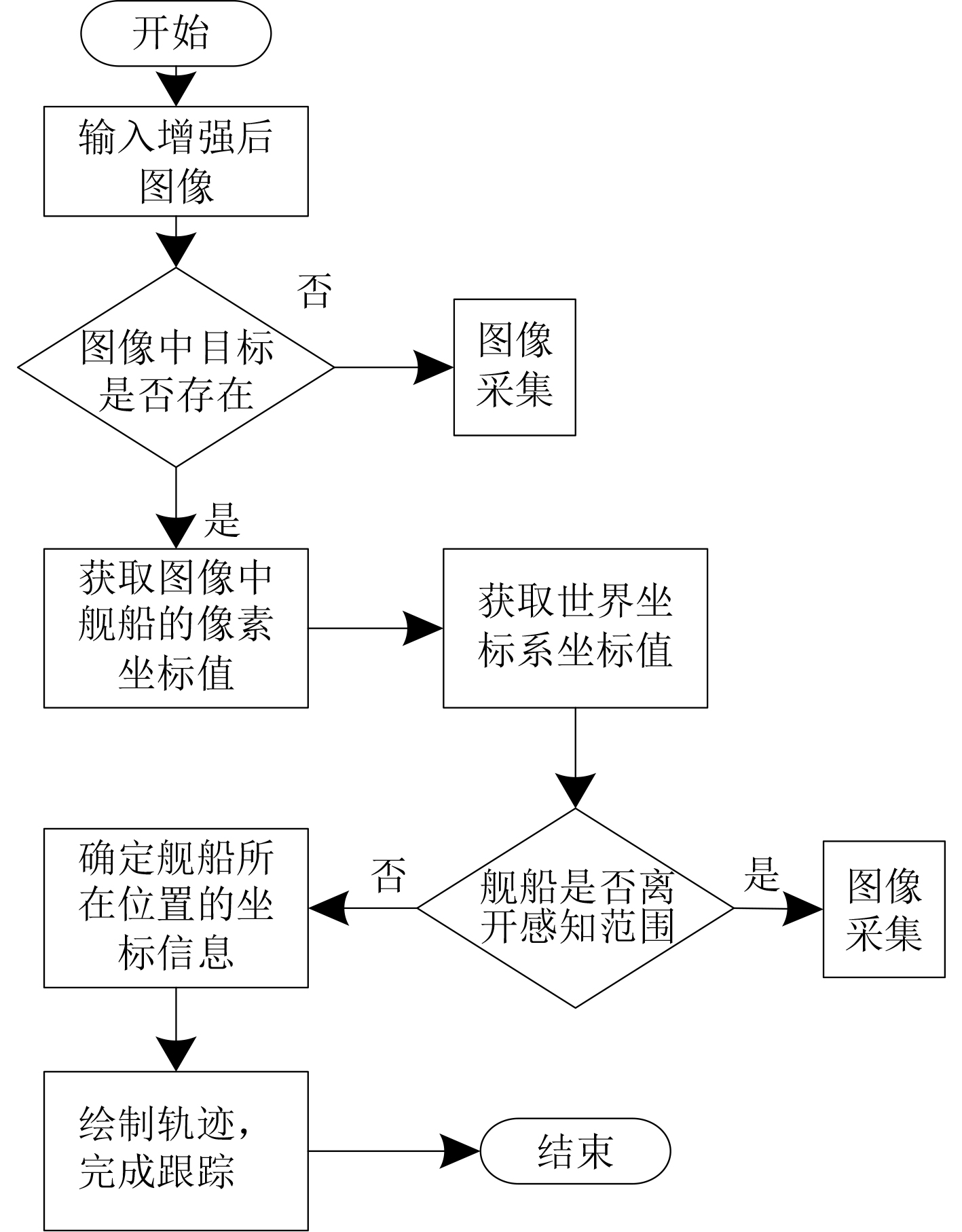图 2 目标跟踪流程 Fig. 2 Target tracking process

 $s\left[ {\begin{array}{*{20}{c}} u \\ v \\ 1 \end{array}} \right] = {{\boldsymbol{P}}_{3 \times 4}}\left[ {\begin{array}{*{20}{c}} {{X_W}} \\ {{Y_W}} \\ {{Z_W}} \\ 1 \end{array}} \right]。$ (6)

 $H{X_i} = \left( {\begin{array}{*{20}{c}} {{h^{1T}}X} \\ {{h^{2T}}X} \\ {{h^{3T}}X} \end{array}} \right) ，$ (7)

$X'$ 采用 $X' = {\left( {x',y',w'} \right)^{\rm{T}}}$ 代替，如果 $w'$ 的取值为1， $\left( {x',y'} \right)$ 表示舰船目标图像上的点，那么得出：

 $X' \times HX = \left( {\begin{array}{*{20}{c}} {y'{h^{3T}}x - x'{h^{2T}}x} \\ {w'{h^{1T}}x - x'{h^{3T}}} \\ {x'{h^{2T}}x - y'{h^{1T}}x} \end{array}} \right) ，$ (8)

 ${{\boldsymbol{A}}_{\rm{i}}}h = 0 ，$ (9)

 $h = \left( {\begin{array}{*{20}{c}} {{h^1}} \\ {{h^2}} \\ {{h^3}} \end{array}} \right),H = \left[ {\begin{array}{*{20}{c}} {{h_1}}&{{h_2}}&{{h_3}} \\ {{h_4}}&{{h_5}}&{{h_1}} \\ {{h_7}}&{{h_8}}&{{h_9}} \end{array}} \right] ，$ (10)

 $\left[ {\begin{array}{*{20}{c}} {{0^{\rm{T}}}}&{ - w'{X^{\rm{T}}}}&{y'{X^{\rm{T}}}} \\ {w'{X^{\rm{T}}}}&{{0^{\rm{T}}}}&{ - x'{X^{\rm{T}}}} \end{array}} \right]\left( {\begin{array}{*{20}{c}} {{h^1}} \\ {{h^2}} \\ {{h^3}} \end{array}} \right) = 0 。$ (11)

1）获取二维平面上的坐标点，其数量为 $n$ ，依据 $X' \times HX = 0$ 得出维数为2×9的 ${{\boldsymbol{A}}_i}$ 矩阵。

2）对Ai实行整理，获取维数为2n×9的矩阵 ${\boldsymbol{A}}$

3）采用奇异值方法对 ${\boldsymbol{A}}$ 实行分解处理，采用奇异值最小的单位奇异向量描述矩阵 $h$ 的结果。

4）依据式(10)即可求解得出单应矩阵 ${\boldsymbol{H}}$ ，即确定舰船的实际位置，实现舰船跟踪。

2 测试分析表 1 图像增强效果测试结果 Tab.1 Test results of image enhancement effect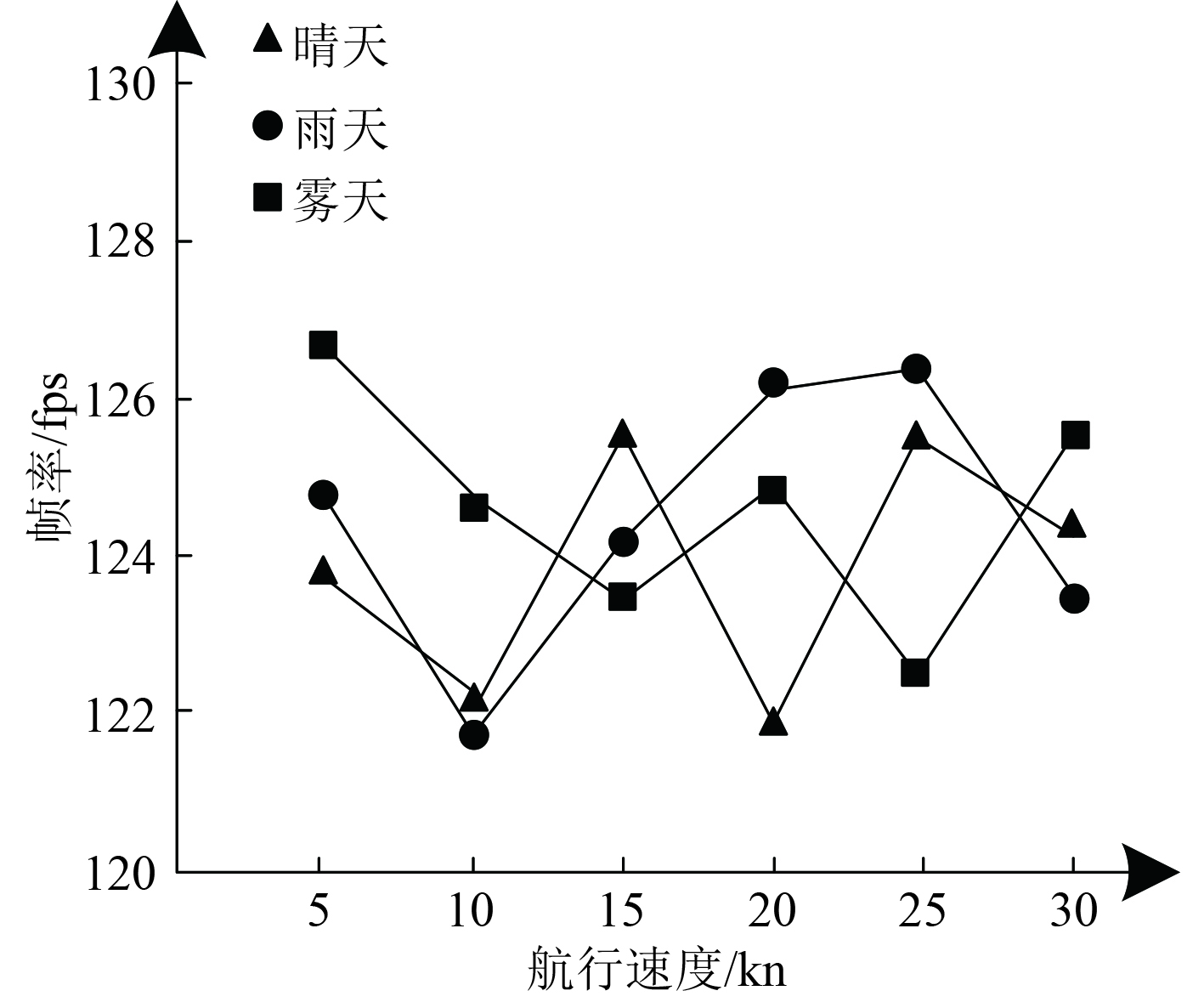图 3 跟踪性能测试结果 Fig. 3 Tracking performance test results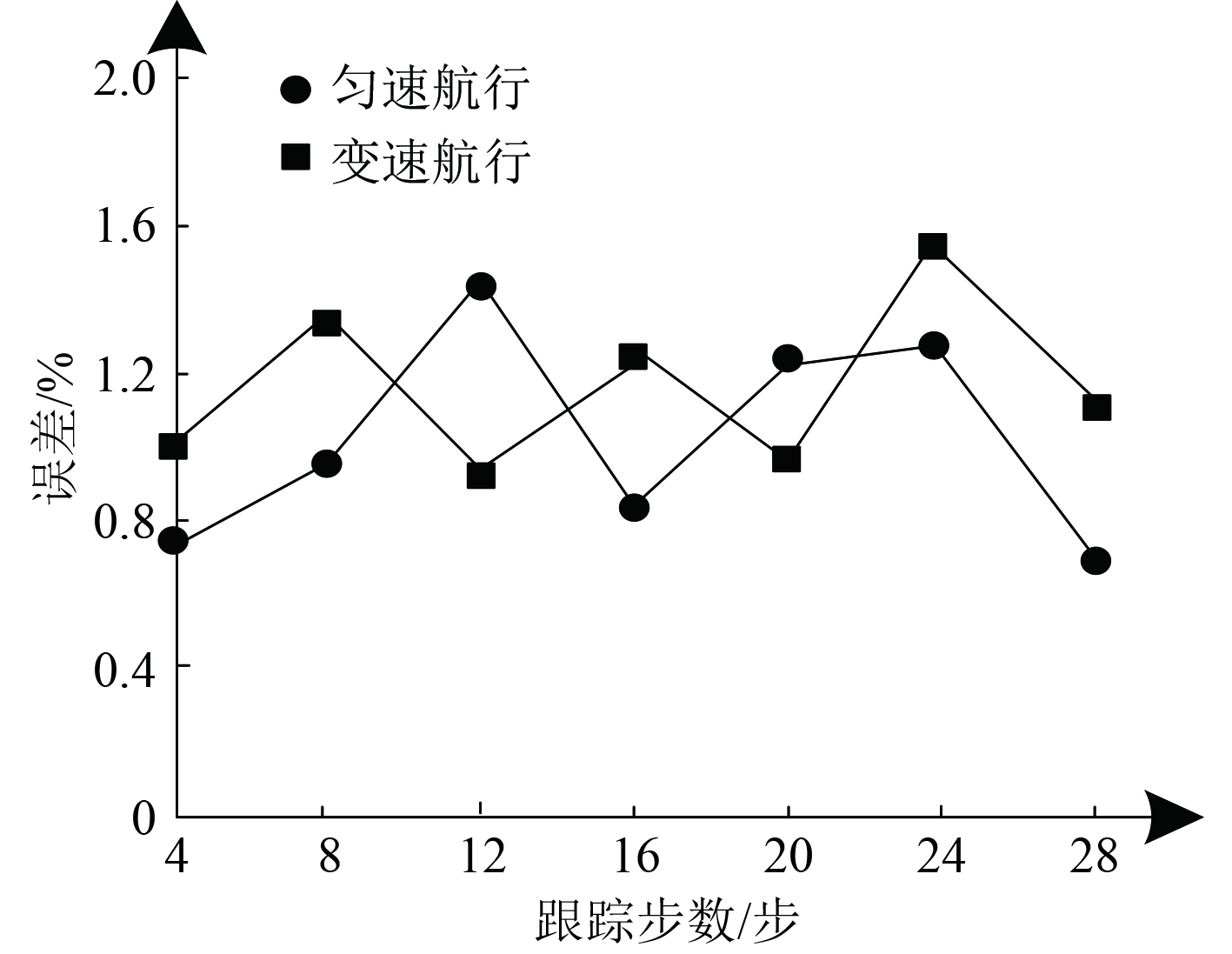图 4 跟踪效果测试结果 Fig. 4 Tracking effect test results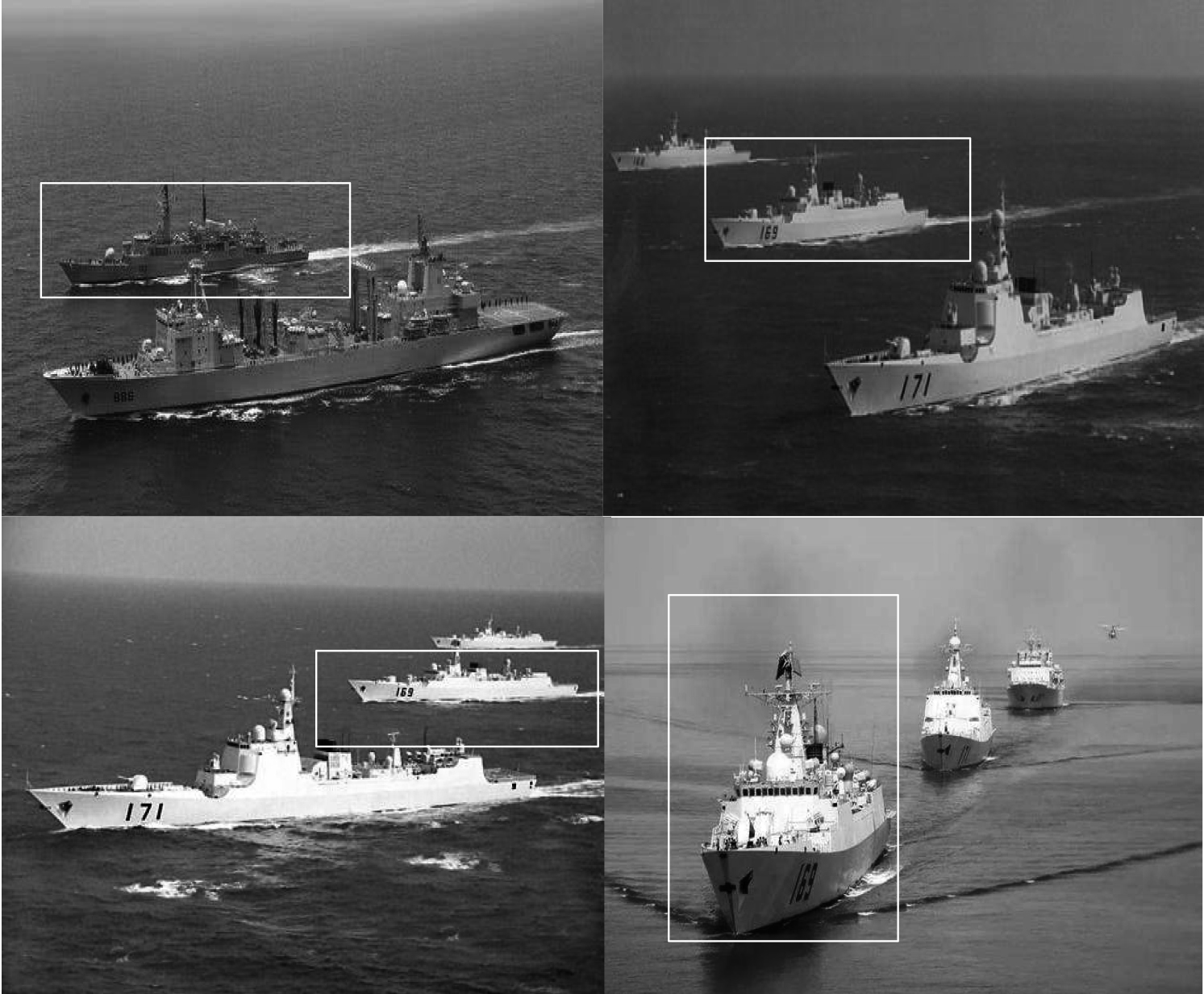图 5 发生遮挡时的跟踪结果 Fig. 5 Tracking results when occlusion occurs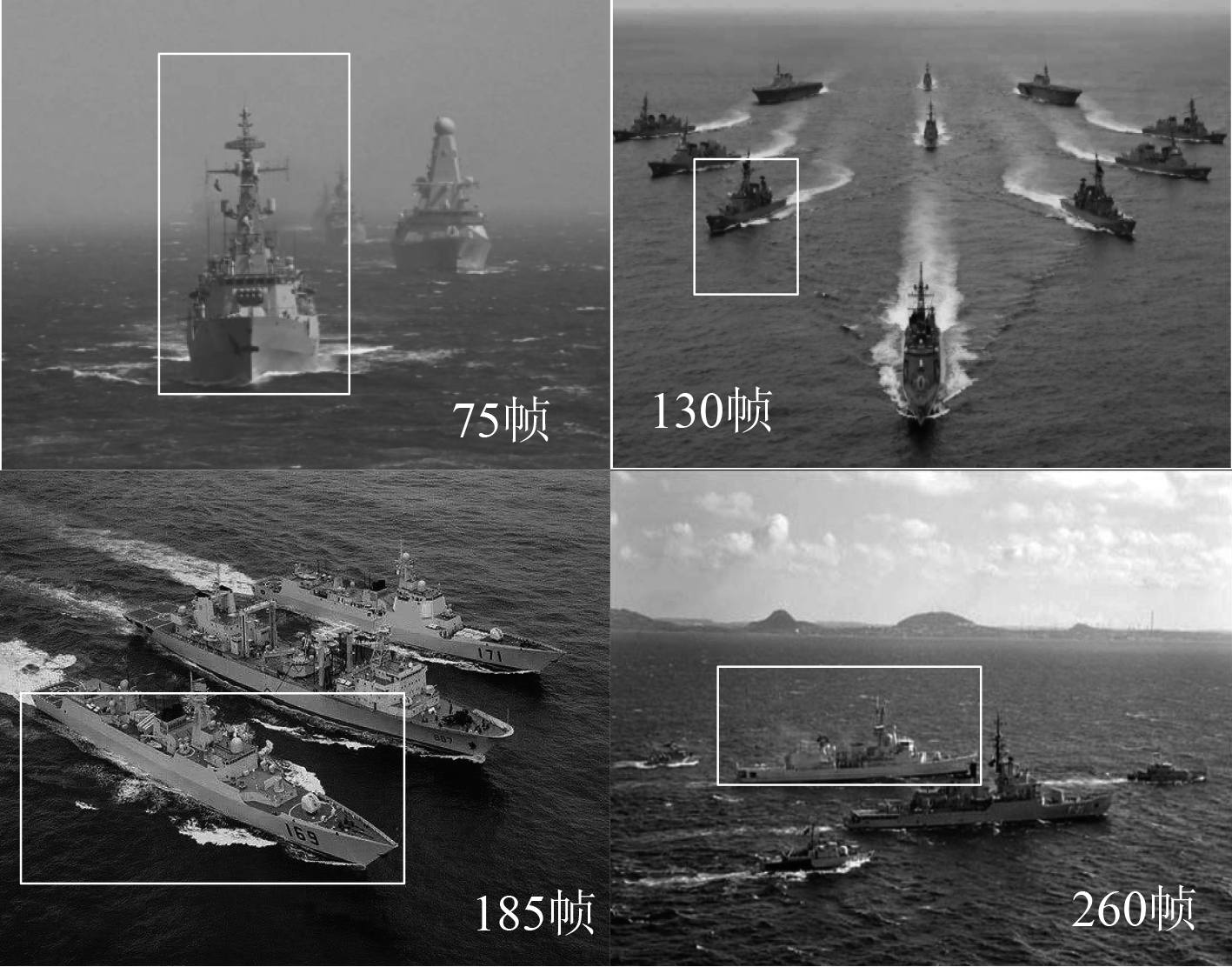图 6 尺度发生变化时的跟踪结果 Fig. 6 Tracking results when scale changes
3 结　语

  孙忠镇, 戴牧宸, 雷禹, 等. 基于级联网络的复杂大场景SAR图像舰船目标快速检测[J]. 信号处理, 2021, 37(6): 941-951.  王国豪, 李怀忠, 马云明. 考虑目标捕获率和能量限制的无线传感器网络管理[J]. 传感技术学报, 2020, 33(3): 464-470. DOI:10.3969/j.issn.1004-1699.2020.03.023  刘子龙, 王晨. 基于双模态输入的孪生网络目标跟踪算法[J]. 计算机应用研究, 2021, 38(12): 3796-3800.  李晓强, 陈建峰, 张蓉蓉, 等. 基于快速正交匹配追踪的无线传感网中目标定位算法[J]. 西北工业大学学报, 2020, 38(1): 31-39. DOI:10.3969/j.issn.1000-2758.2020.01.005  赵钦炎, 李宗民, 刘玉杰, 等. 基于信息熵的级联Siamese网络目标跟踪[J]. 计算机科学, 2020, 47(9): 157-162. DOI:10.11896/jsjkx.190800160  张伽伟, 喻鹏, 姜润翔, 等. 基于舰船电场的目标跟踪方法研究[J]. 兵工学报, 2020, 41(3): 559-566. DOI:10.3969/j.issn.1000-1093.2020.03.017  刘明珠, 苏桃宇, 王依宁. 压扩多尺度Retinex图像增强算法研究[J]. 哈尔滨理工大学学报, 2020, 25(5): 93-99.  蒲恬, 张子夜, 彭真明. 保持自然度的非均匀光照图像增强的Retinex算法[J]. 数据采集与处理, 2021, 36(1): 76-84.  骆家杭, 张旭. 基于改进型Retinex算法的彩色图像增强技术[J]. 计算机工程与科学, 2021, 43(5): 891-896. DOI:10.3969/j.issn.1007-130X.2021.05.016